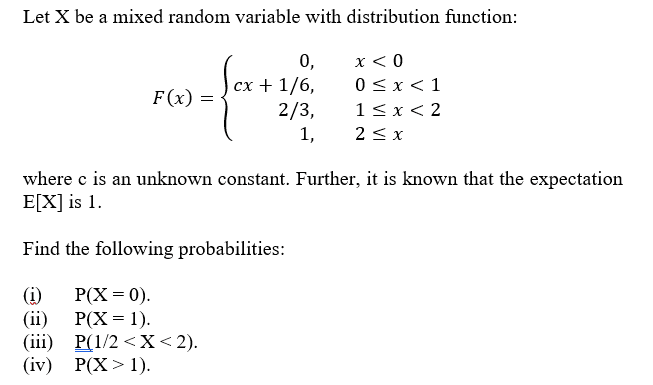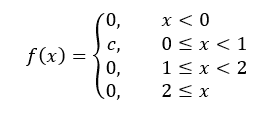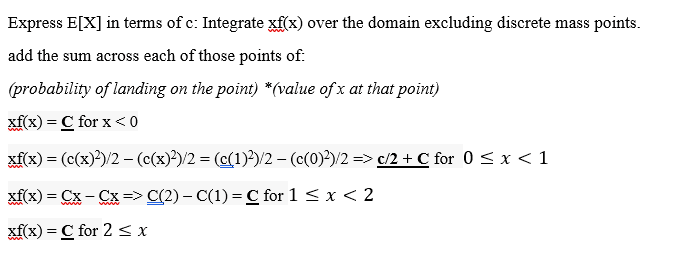# Mixed random variable distribution question

## Homework Statement

See attached image (See below)

## Homework Equations

Differential equations.
And a combination of discrete & continuous distributions

## The Attempt at a Solution

The Continous Distribution Function (CDF) is given in the question. So I differentiated it with respect to x piecewise (See below). That is what I got

I can also see that there is a uniform distribution between 0 and 1 but also there are discrete points that have assigned probability. For example; those points could be 0,1 and 2? maybe?
I want to be able to find the value of c while remembering the sum of the probabilities must add up and equal to 1.

How do I go about solving for c? this is where I am stuck. I cannot seem to figure out where to go from here. Thanks!

Question:(When I differentiated it)

#### Attachments

andrewkirk
Homework Helper
Gold Member
The calculation of the density function ##f## is not quite correct. The density is infinite at ##x=0,2## and also possibly at 1 (depending on the value of ##c##), because the CDF jumps up discontinuously at those points. We say there is a 'discrete probability mass' at those infinite-density points, meaning there is a non-zero probability of X having exactly that value.

You need to use the additional fact that ##E[X]=1##. Using the formulas you got for ##f##, corrected to also include the points of discrete probability mass at 0 and 2, write an expression for ##E[X]## in terms of ##c##. Equating that expression to 1 will give you an equation you can solve to find ##c##. The expression will be a sum of integrals and isolated discrete terms.

There are two cases to consider here - (1) where there is a discrete probability mass at ##x=1## and (2) where there is not. I suggest trying case (1) first. If that does not give an acceptable solution, try case (2).

•King_Silver
The calculation of the density function ##f## is not quite correct. The density is infinite at ##x=0,2## and also possibly at 1 (depending on the value of ##c##), because the CDF jumps up discontinuously at those points. We say there is a 'discrete probability mass' at those infinite-density points, meaning there is a non-zero probability of X having exactly that value.

You need to use the additional fact that ##E[X]=1##. Using the formulas you got for ##f##, corrected to also include the points of discrete probability mass at 0 and 2, write an expression for ##E[X]## in terms of ##c##. Equating that expression to 1 will give you an equation you can solve to find ##c##. The expression will be a sum of integrals and isolated discrete terms.

There are two cases to consider here - (1) where there is a discrete probability mass at ##x=1## and (2) where there is not. I suggest trying case (1) first. If that does not give an acceptable solution, try case (2).

Thank you for that. I figured it might have had something to do with the expectation formula. E[X] = 1 in terms of c. There are definitions for the expectation of continuous random variables and for discrete ones as well. For a mixed distribution you would need to mix both definitions together right?
I'm having a lot of trouble trying to figure out how to mix these two expectation formulas

andrewkirk
Homework Helper
Gold Member
I'm having a lot of trouble trying to figure out how to mix these two expectation formulas
Just integrate ##xf(x)## over the domain excluding the discrete mass points, and add the sum across each of those points of (probability of landing on the point) x (value of x at that point).

Just integrate ##xf(x)## over the domain excluding the discrete mass points, and add the sum across each of those points of (probability of landing on the point) x (value of x at that point).

This is what I did but I don't think I'm going in a correct direction. C = constant whereas c = the value i'm looking for.Ray Vickson
Homework Helper
Dearly Missed

## Homework Statement

See attached image (See below)

## Homework Equations

Differential equations.
And a combination of discrete & continuous distributions

## The Attempt at a Solution

The Continous Distribution Function (CDF) is given in the question. So I differentiated it with respect to x piecewise (See below). That is what I got

I can also see that there is a uniform distribution between 0 and 1 but also there are discrete points that have assigned probability. For example; those points could be 0,1 and 2? maybe?
I want to be able to find the value of c while remembering the sum of the probabilities must add up and equal to 1.

How do I go about solving for c? this is where I am stuck. I cannot seem to figure out where to go from here. Thanks!

Question:
View attachment 213731 View attachment 213732(When I differentiated it)

For a non-negative random variable ##X##, whether continuous, discrete or mixed, if ##F(x) = P(X \leq x)## is the CDF and ##G(x) = P(X > x) = 1 - F(x)## is the complementary CDF, then we have that
$$EX = \int_0^{\infty} G(x) \, dx$$
You can do the integral in terms of ##c## and so get an equation for ##c##.

•StoneTemplePython and King_Silver
For a non-negative random variable ##X##, whether continuous, discrete or mixed, if ##F(x) = P(X \leq x)## is the CDF and ##G(x) = P(X > x) = 1 - F(x)## is the complementary CDF, then we have that
$$EX = \int_0^{\infty} G(x) \, dx$$
You can do the integral in terms of ##c## and so get an equation for ##c##.

I got a c value of 1/3 by doing this (1 = c/2 + ½ -c + 2/3 )
Then for part (i): Probability = 1/6th or 16.67%
(ii) Probability = 2/3rds or 66.67%
(iii) Probability = 1/3rd or 33.3%
(iv) Probability = 1/2 or 50%

I think I've got it now providing those are the right answers!

Ray Vickson
Homework Helper
Dearly Missed
I got a c value of 1/3 by doing this (1 = c/2 + ½ -c + 2/3 )
Then for part (i): Probability = 1/6th or 16.67%
(ii) Probability = 2/3rds or 66.67%
(iii) Probability = 1/3rd or 33.3%
(iv) Probability = 1/2 or 50%

I think I've got it now providing those are the right answers!

The first two are; I have not checked the other two.

However, in English, "1/6" is already pronounced as "one-sixth", so when you write 1/6th you are really writing "one-sixth-th". Thus, the The first two answers are just 1/6 and 2/3 (not "two-thirds-irds").

Last edited: